﻿ 电子商务增长模型的分析预测 Analysis and Prediction of Electronic Commerce Growth Model

Statistics and Application
Vol. 08  No. 01 ( 2019 ), Article ID: 28957 , 8 pages
10.12677/SA.2019.81020

Analysis and Prediction of Electronic Commerce Growth Model

Hongzhan Chen, Jing Zheng

The College of Economics, Hangzhou Dianzi University, Hangzhou Zhengjiang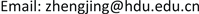Received: Feb. 2nd, 2019; accepted: Feb. 14th, 2019; published: Feb. 21st, 2019ABSTRACT

Based on Chinese quarterly e-commerce transactions data in recent years, the quadratic, the exponential and the logistic growth models are fitted. Using MATLAB software, we plot the e-commerce growth data points as well as the forecast figure, and estimate the model parameters, goodness-of-fit statistics and model residual analysis. The analytic model of the fitting curve and forecast for the future scale of e-commerce data are given.

Keywords:Electronic Commerce, Logistic Growth Model, Residual AnalysisCopyright © 2019 by author(s) and Hans Publishers Inc.1. 引言

2. 模型介绍

2.1. 二次函数增长模型

$x\left(t\right)=a{t}^{2}+bt+c$

2.2. 指数函数增长模型

2.3. 阻滞增长模型

$\frac{\text{d}x}{\text{d}t}=r{\left(1-\frac{x}{{x}_{m}}\right)}^{x},x\left(0\right)={x}_{0}$

$x\left(t\right)=\frac{{x}_{m}}{1+\left(\frac{{x}_{m}}{x\left(0\right)}-1\right){\text{e}}^{-rt}}$

3. 实证检验

3.1. 模型的描述统计Table 1. E-commerce transaction data in quarter from 2010 to 2016

3.2. 三个模型的拟合结果描述

3.3. 三条曲线的拟合曲线及预测图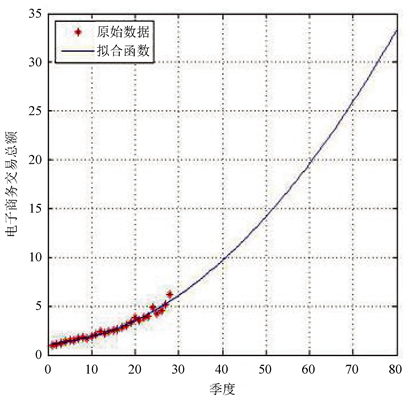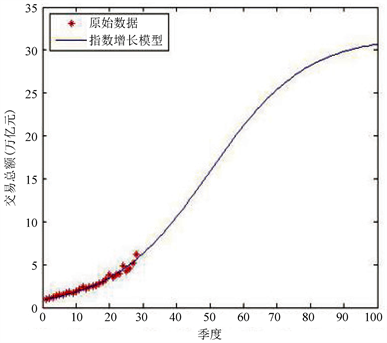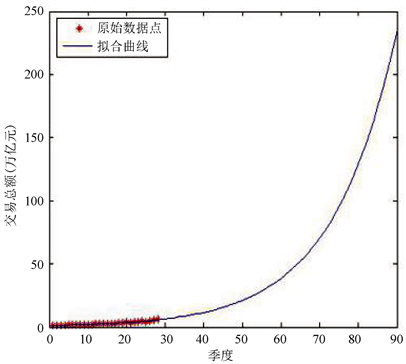Figure 1. Raw data and fit curve

4. 模型的残差分析

4.1. 自相关分析检验残差的独立性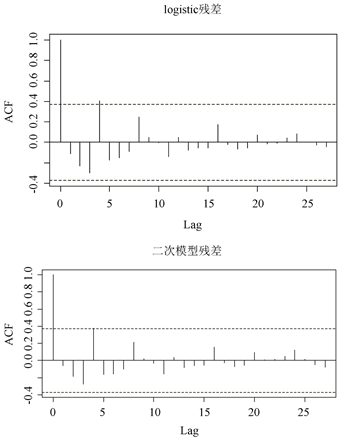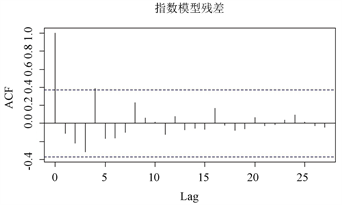Figure 2. Residual autocorrelation coefficient

0的独立同分布随机变量序列。从图中我们可以得出残差项存在独立性。

4.2. 残差的正态分布检验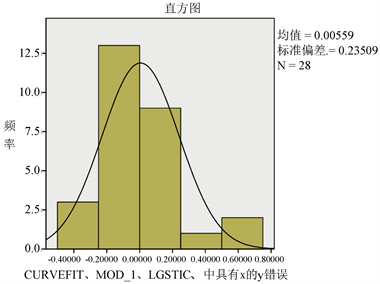Figure 3. Histogram of residuals

4.3. 未来季度数据预测

5. 结束语

Analysis and Prediction of Electronic Commerce Growth Model[J]. 统计学与应用, 2019, 08(01): 176-183. https://doi.org/10.12677/SA.2019.81020

1. 1. 刘江伟, 于立. 电子商务生态系统文献综述及评价[J]. 科技创新与生产力, 2017(9): 31-33.

2. 2. 吴嘉伟. “互联网+”时代的电子商务[J]. 现代企业文化, 2017(21): 221.

3. 3. 翟凤荣. 我国电子商务现状及展望[J]. 烟台大学学报哲学社会科学版, 2000, 13(4): 451-456.

4. 4. 王丽莉. 我国电子商务现状及未来发展趋势[J]. 网络与信息, 2008(4): 30.

5. 5. 资道根. 基于灰色GM(1,1)模型的跨境电子商务发展前景预测分析[J]. 数学的实践与认识, 2015, 45(1): 96-105.

6. 6. Matis, J.H. and Kiffe, T.R. (2004) On Stochastic Logistic Population Growth Models with Immigration and Multiple Births. Theoretical Population Biology, 65, 89-104. https://doi.org/10.1016/j.tpb.2003.08.003

7. 7. 黄润龙, 帅友良. 人口增长的Logistic模型及其应用研究[J]. 人口与社会, 2000, 16(3): 25-27.

8. 8. 代涛, 徐学军, 黄显峰. 离散Logistic人口增长预测模型研究[J]. 三峡大学学报(自然科学版), 2010, 32(5): 102-105.

9. 9. 魏金岭, 赵光宙. Logistic人口增长模型的反馈控制研究[J]. 系统工程理论与实践, 1999, 19(11): 115-120.